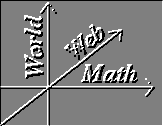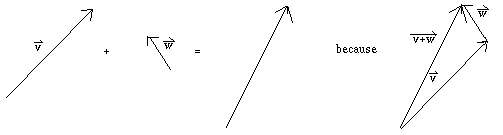#Vector Arithmetic

Pre-requisites: Vectors

One of the differences between vectors and points is that while it doesn't really make sense to add or subtract points, it does make sense to add and subtract vectors. Here's how:

To add two vectors v and w, we consider v as the displacement vector OP for some P and then w as the displacement vector PQ for some Q. The answer, v + w is then the displacement vector OQ.This definition of vector arithmetic has the singular advantage that it agrees with nature: it is a physical law that if a particle is being acted on by two forces F1 and F2, the result is the same as if the particle were only being acted on by the one force F1 + F2 .

The following picture proves that vector addition is commutative; i.e., that v + w = w + v : [Image of "Parallelogram Law"]

To define how to subtract two vectors, it suffices to define how to negate a single vector, as v - w = v + ( - w ) . This is easy enough: -v is simply the vector with the same magnitude as v , but with the opposite direction. To put it another way, if v = OP for some point P, then -v = PO.

Finally, we can define how to multiply a vector by a scalar real number: c v for some non-negative real number c is the vector with the same direction as v, but with c times v's magnitude. This definition of scalar multiplication has all of the properties you would expect it to have: v + v = 2 v and more generally a v + b v = (a+b) v .

Fortunately, all of these operations are easy to calculate in Cartesian coordinates. The trick is to realize that (v1, v2, v3) and v1 i + v2 j + v3 k are the same vector. Why? Because they have the same projections onto the coordinate axes. Once we have this knowledge, we can see that ( v1, v2, v3 ) + ( v4, v5, v6 ) = v1 i + v2 j + v3 k + v4 i + v5 j + v6 k = ( v1 + v4 ) i + ( v2 + v5 ) j + ( v3 + v6 ) k = ( v1 + v4, v2 + v5, v3 + v6 ) , or that coordinate-wise, vector addition is the same as tuple addition.

It should then come as no surprise that multiplying a vector by a scalar is the same as multiplying a tuple by a scalar: c ( v1, v2, v3 ) = ( c v1, c v2, c v3 ) . To prove this, let ( v1, v2, v3 ) = OP for some point P and let c ( v1, v2, v3 ) = OQ for some point Q. By hypothesis, O, P, and Q all lie on the same line. If we project P and Q onto the XY plane and call the results P' and Q respectively, then we get two similar triangles: tri(O P P') and tri(O Q Q'). We are given that segment(OQ) = c segment(OP) , so by the Similar Triangles Theorem segment(Q Q') = c segment(P P') . But segment(P P') = v3 , so the Z component of c ( v1, v2, v3 ) must be c v3. The same reasoning holds for the X and Y components, so the result follows.

### Exercises:

1. Prove that vector addition is associative; i.e, that u + ( v + w ) = ( u + v ) + w .

2. Prove that v + ( - v ) = 0 .

3. Prove that c ( d v ) = ( c d ) v .

4. Prove that c 0 = 0 .

5. Prove that 0 v = 0 .

6. Show that OP - OQ = QP .

7. In the following window, draw in
1. the sum of the red and blue vector
2. the red vector minus the blue vector
3. three times the blue vector

8. Prove that c ( v + w ) = c v + cv .

Solutions to Exercises | Vector Calculus Index | World Web Math Main Page

thomasc@athena.mit.edu